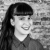# How do I display the value of a variable ( percentage completed ) ?

Known Prototypo ago

## So I'm creating a tutorial and I want to display the percentage completed on each page. How can I do that? So far I have three variable - page_number, perc_calc and progress. The perc calc has a value of the total number of pages and the progress variable is basically page_number / perc_calc to give a decimal percentage completed. How do I do that and how do I display the value of 'progress'?

Replies (1)Hi there,

An option would be creating a set value event of a variable that contains and controls de "page number". Then an "on page load" / "set value" with an associated calculated expression that divides the Variable / Total amount of pages and another "on page load" event that adds +1 to the variable.

Best,

Sonia Durán